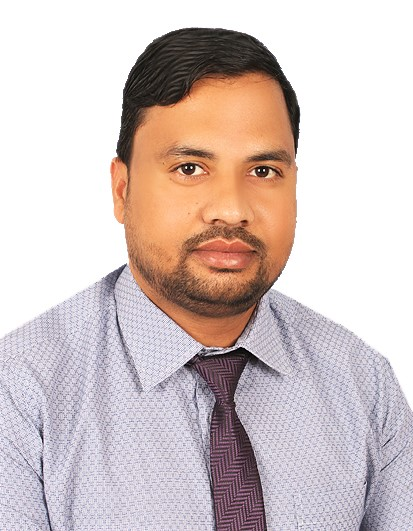|   December 02, 2023DR. SUNIL KUMAR MAURYA
Assistant Dean for Graduate Studies & Research & Associate Professor
Dean Office, Mathematical and Physical Sciences - Mathematics Section
College of Arts and Sciences
• Telephone: (+968)25446200
• Extension: 943
• eMail: sunil@unizwa.edu.om
• Office Location: 5D-5A
• Marital Status: ...

• Received Ph.D. on 22/03/2013 from IIT Roorkee (India) in Mathematics, M.Sc. in Mathematics from the University of B.H.U. (India) in 2008, and B.Sc. in Maths & Physics from the University of Lucknow (India) in 2006. His research interests are- Differential equations, General Relativity, Cosmology, Similarity transformations (Lie group of transformations).

• Ph.D, IIT Roorkee, 2013
Teaching Activities
• Engineering Mathematics-I, Differential Calculus, Integral Calculus, Ordinary differential equations and Fourier series
• Engineering Mathematics-III:, Matrix, Vector space and Complex analysis.
• Calculus-II, hyperbolic, inverse trigonometric functions, techniques of integration, Applications of integration (volumes, surfaces, arc-length), the definite integral, sequences and series, Taylor polynomials and approximation.
• Calculus-I, Limits, Differentiation, Integrations
• Precalculus
• Differential Equations
• Mathematical Economics : , derivatives and optimization techniques for functions, basic techniques of integration, definite integrals. The emphasis is placed on applications of derivatives and integrals to various problems from business, economics and finance.
• Foundation of Mathematics, Logic and proofs, Sets, Relations, Equivalence Relations, Functions and Cardinality.
• Foundation of Analysis, : field axioms, ordered axioms, Supremum and Infimum, Completeness of , Properties of Supremum and Infimum (Proofs of Uniqueness, Approximation, Monotonicity, and Additive Properties, and other properties) , and Archimedean property of real numbers; The dense set. The sets of rational numbers and of irrational numbers are dens in the set of real numbers
• Introduction to Number theory, integers, divisibility, primes and their distribution, the theory of congruences, Fermat`s and Euler theorems, and applications
• Mathematical Economics for Masters, statics (equilibrium analysis), comparative statics, and optimization problems and dynamics, making use of the following mathematical methods: differential and integral calculus (advanced calculus), dynamic optimization, linear programming and differential equations. They will be able to select and apply appropriate techniques to solve problems apply mathematical and graphical techniques in an appropriate manner.
• Engineering Mathematics-II:, Vector calculus, Partial Differential Equations and Laplace transforms
Research Activities
- Research Interests
• Modelling of compact stars, Astronomy & Astrophysics
• Differential Equations
• General Relativity and Cosmology
• Exact solution of Einstein-field Equations
- Publications
Membership in Professional Bodies
• 2014-Present: Editorial Board Member of the International Journal of Modern Physics and Application.
• 2014-Present: Member of International Association of Computer Science and Information Technology (IACSIT)